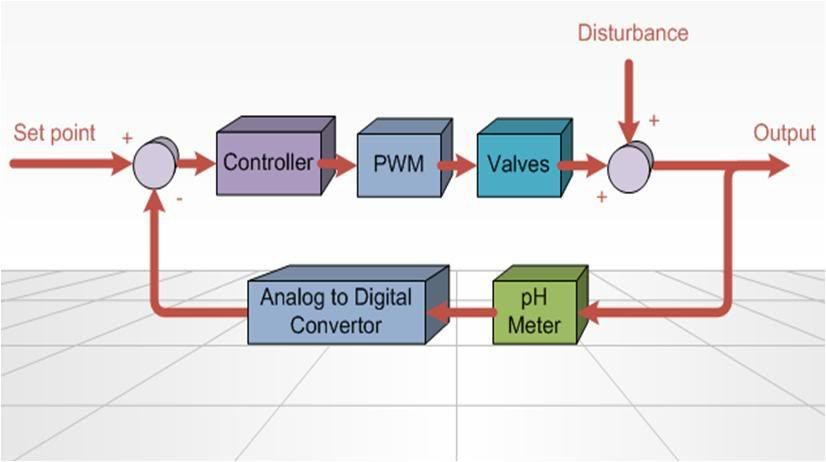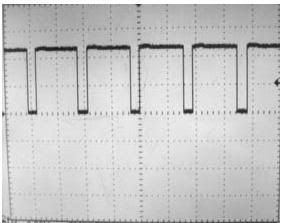Control System

## The Control SystemThe purpose of the feedback control is the help the system reach the set point as fast as possible. Figure above shows the negative feedback control system with all the components included. The sensor being the pH meter constantly feedback to the set point indicating whether the system has reached a steady state condition. The controller which is going to be implemented using algorithm inside the microcontroller is the proportional integral (PI) controller.

The PIC will then process the information and control the actuators (in this case is valves) using Pluse Width Modulation (PWM) to release acid or base into the solution to achieve the desired set point. Along the way there could be disturbance which will affect the accuracy of the controller. At the end of the feedback control, there will be an output which is the current pH of the solution containing the red cabbage.

## **Proportional Integral Controller **

The PI controller is implemented by my FYP partner Weicong. Different parameters of the controller will affect the performance of the control system, so this portion is included to show how the different parameter plays a part inside the control equation.

Control Equation in S domain:
M_t= (K_p+ 1/s K_i ) E_t —— (1)

M_t= (K_p+ 1/s K_i ) E_t —— (1)
M_t is the output during time t E_t is the error during time t
K_p is the proportional Constant K_i is the integral constant

Using Bilinear Transformation:
s= 2/T (z-1)/(z+1)= 2/T (1- z^(-1))/(1+ z^(-1) ) —— (2)
T is the sampling time

Hence by substituting (2) into (1),

M_k= (K_p+ T/2 (1+z^(-1))/(1-z^(-1) ) K_i ) E_k
2(1-z^(-1) ) M_k= [2(1-Z^(-1) ) K_p+T(1+Z^(-1) ) K_i ] E_k
2(M_k-M_(k-1) )=2K_p E_k- 2K_p E_(k-1)+TK_i E_k+TK_i E_(k-1)
M_k=1/2 [(TK_i+2K_p ) E_k+(TK_i-2K_p ) E_(k-1) ]+M_(k-1)

M_k is the output during time period k
M_(k-1) is the output during time period k-1
E_k is the error during time period k
E_(k-1) is the error during time period k-1

## Pulse Width ModulationThe pumps that we are using can only be either turn on or off. In order to control the pumps more accurately, we need to use Pluse Width Modulation (PWM). PWM is basically turning on a device for a certain amount of time during a period. The figure above illustrates the PWM working at 80% duty cycle. The frequency that we are using for the PWM is 40Hz.

page revision: 1, last edited: 22 Apr 2008 04:26